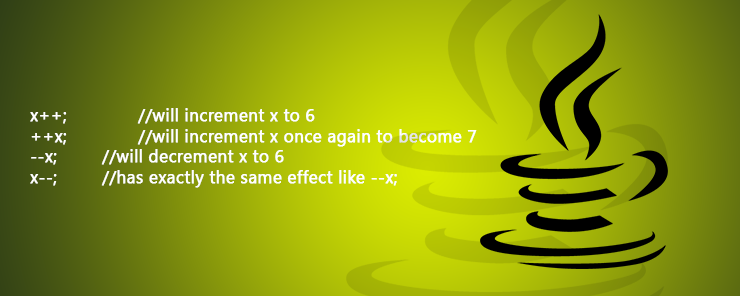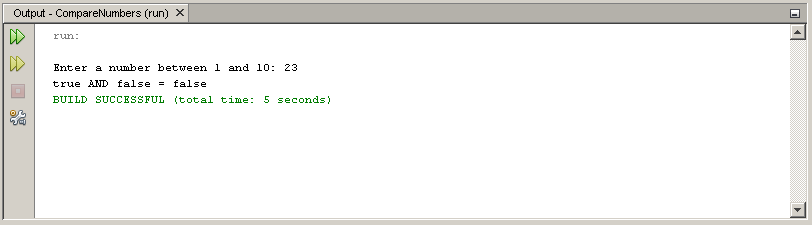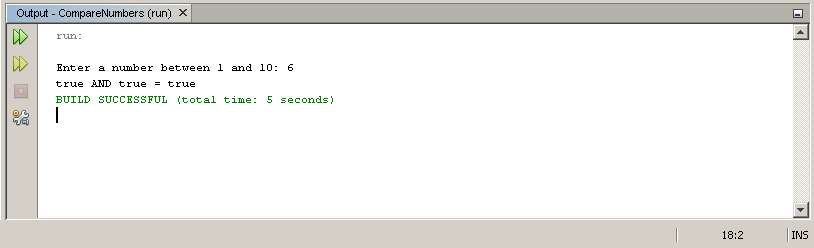# Learn about Operators in Java Programming

0
3618As I told you in the conclusion part of the previous article: After learning the variables and data types, we should know what to do with those variables. Operators are going to give us the answer.

What are Operators?
In a very short and descriptive definition: Operators help us manipulate data stored in variables.
As in other programming languages, Java supports several types of operators: Arithmetic operators, Increment/Decrement operators, Assignment operators, Comparison operators, and Logical operators.

Arithmetic Operators
As their name suggests, arithmetic operators perform the basic arithmetic operations.

 Operator Function + Adds two numbers. – Subtracts the right side operand from the left side one. * Multiplies two numbers. / Divides the left side operand by the right side one. % Divides the left side operand by the right side one and returns the remainder.

Examples

```total = a + b;
product = a * b;
result = a / b;
average = (a +b) / 2;
```

Increment / Decrement Operators
Consider the case when we need to increment or decrement a variable by one. This can be easily achieved using the Increment operators ++ and the Decrement operator —

```counter++;		//this increments the variable counter by 1
counter--; 	//this decrements the variable counter by 1
```

The above statements are equivalent to saying:

```counter = counter + 1;
counter = counter - 1;
```

Post and Pre Increment / Decrement
Both the increment ++ and the decrement — operators can be used before or after the variable. In a case like the above one, using the operator before or after the variable won’t make a difference.

Given that x = 5:

```x++;		//will increment x to 6
++x;		//will increment x once again to become 7
--x; 		//will decrement x to 6
x--;		//has exactly the same effect like --x;
```

To understand the difference, consider the following statements starting from x=5:

```y = x++; 		//assign then increment –-> y=5 and x=6
y = ++x;		//increment then assign --> x=7 and y=7
y = x--;		//assign then decrement --> y=7 and x=6
y = --x; 		//decrement then assign --> x=5 and y=5
```

I think it is clear now.

Assignment Operators
Besides to the normal assignment operator = , Java has the following list of assignment operators:

 Operator Function += Adds the right side operand to the left side one, and stores the result in the left side operand. -= Subtracts the right side operand from the left side one, and stores the result in the left side operand. *= Multiplies the two operands and stores the result in the left side one. /= Divides the left side operand by the right side one, and stores the result in the left side operand. %= Calculates the remainder of the division operation and assigns it to the left side operand.

Operators of this type are useful when the value of a variable is being manipulated and the result should be assigned to that variable. Consider the following case:

`total = total + x;`

Using the assignment operators, this statement could be re-written as follows:

`total += x;`

Comparison Operators
The Comparison operators are essential for Decision Making. Expressions that use comparison operators are called Relational Expressions. Their result is either true or false.

 Operator Function < Returns true if the left side operand is less than the right side one. <= Returns true if the left side operand is less than or equal to the right side one. > Returns true if the left side operand is greater than the right side one. >= Returns true if the left side operand is greater than or equal to the right side one. == Returns true if both operands are equal. != Returns true if the two operands are not equal.Logical Operators
Two or more relational expressions can be combined using the Logical Operators. Java supports three basic logical operators that represent the software-developing equivalent to the three basic Logic Gates: AND, OR, and NOT.

 Operator Function && The logical AND operator. It returns true only if both operands are true. || The logical OR operator. It returns true if any of its operands is true. ! The logical NOT operator. It inverts the value of its operand. If the input is true, the output will be false, and vice versa.

Example
The following program will prompt the user to enter a number between 0 and 10. The program will print true if the input is in the specified range. If not, the program will print false.

```import java.util.Scanner;
public class CompareNumbers
{
public static void main(String[] args)
{
System.out.print("\nEnter a number between 1 and 10: ");
float num = new Scanner(System.in).nextFloat();
boolean c1 = num > 0;
boolean c2 = num <=10;
System.out.println(c1 + " AND " + c2 + " = " + (c1 && c2));
}
}
```

When executed, the program should behave like the following:And if the number is in the correct range:String Concatenation Operator
Besides its function as an Arithmetic operator for Addition, the + operator can be used to concatenate two strings together.

Example
The following program concatenates two String variables and stores the resulting string in a third variable.

```public class StringConcat
{
public static void main(String []args)
{
String firstname = "Mohamed";
String lastname = "Ali";
String fullname = firstname + " " + lastname;
System.out.println("Full Name: " + fullname);
}
}
```

When executed it should print the message: Full Name: Mohamed AliSummary